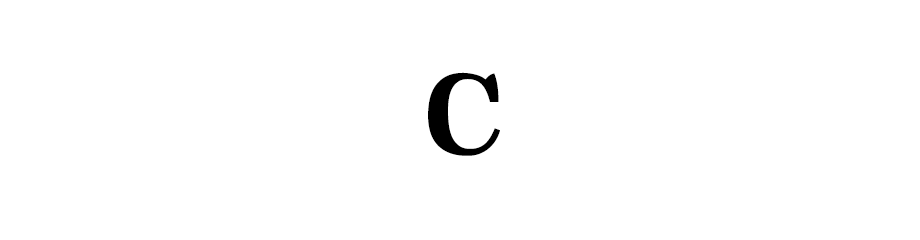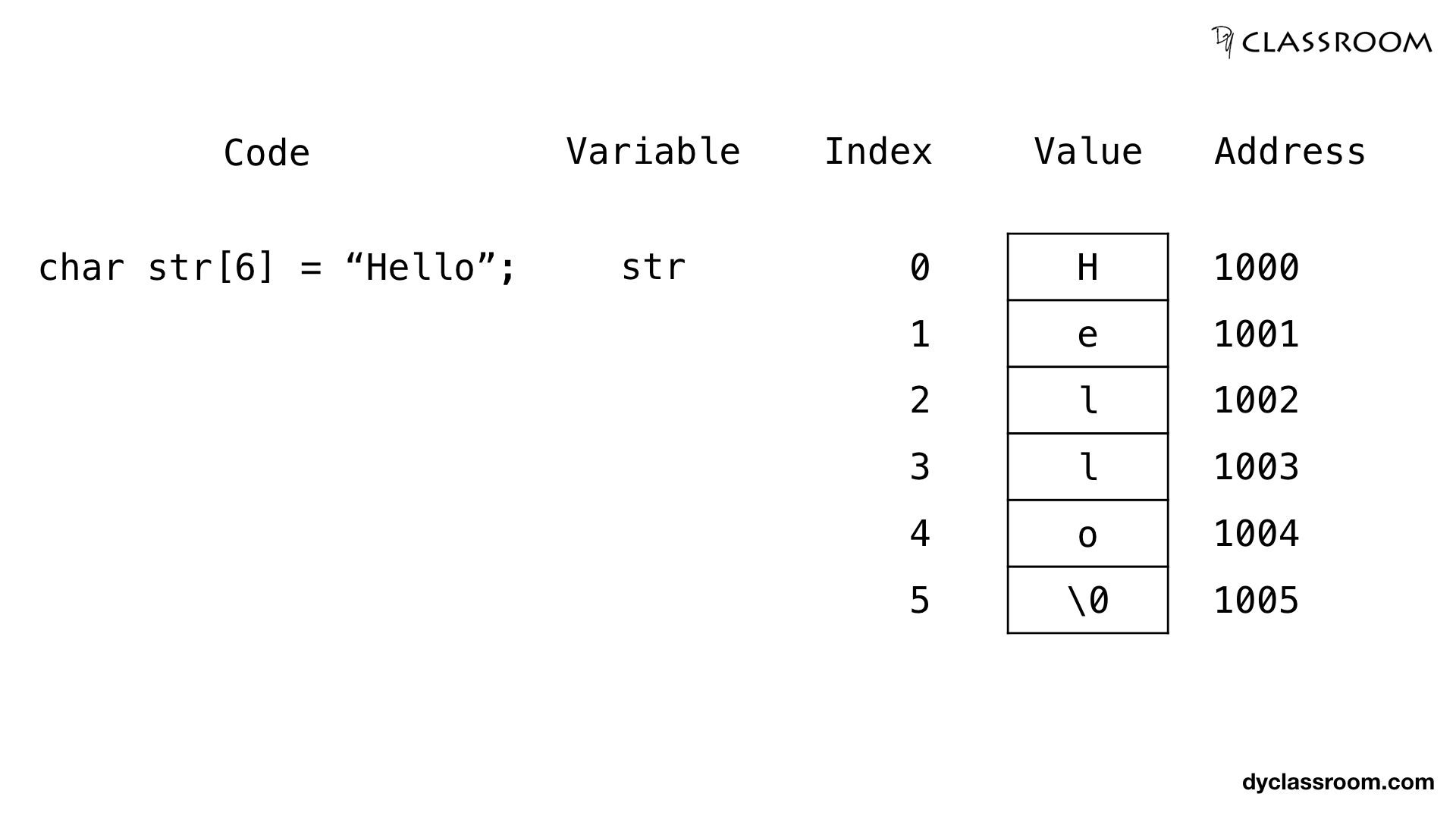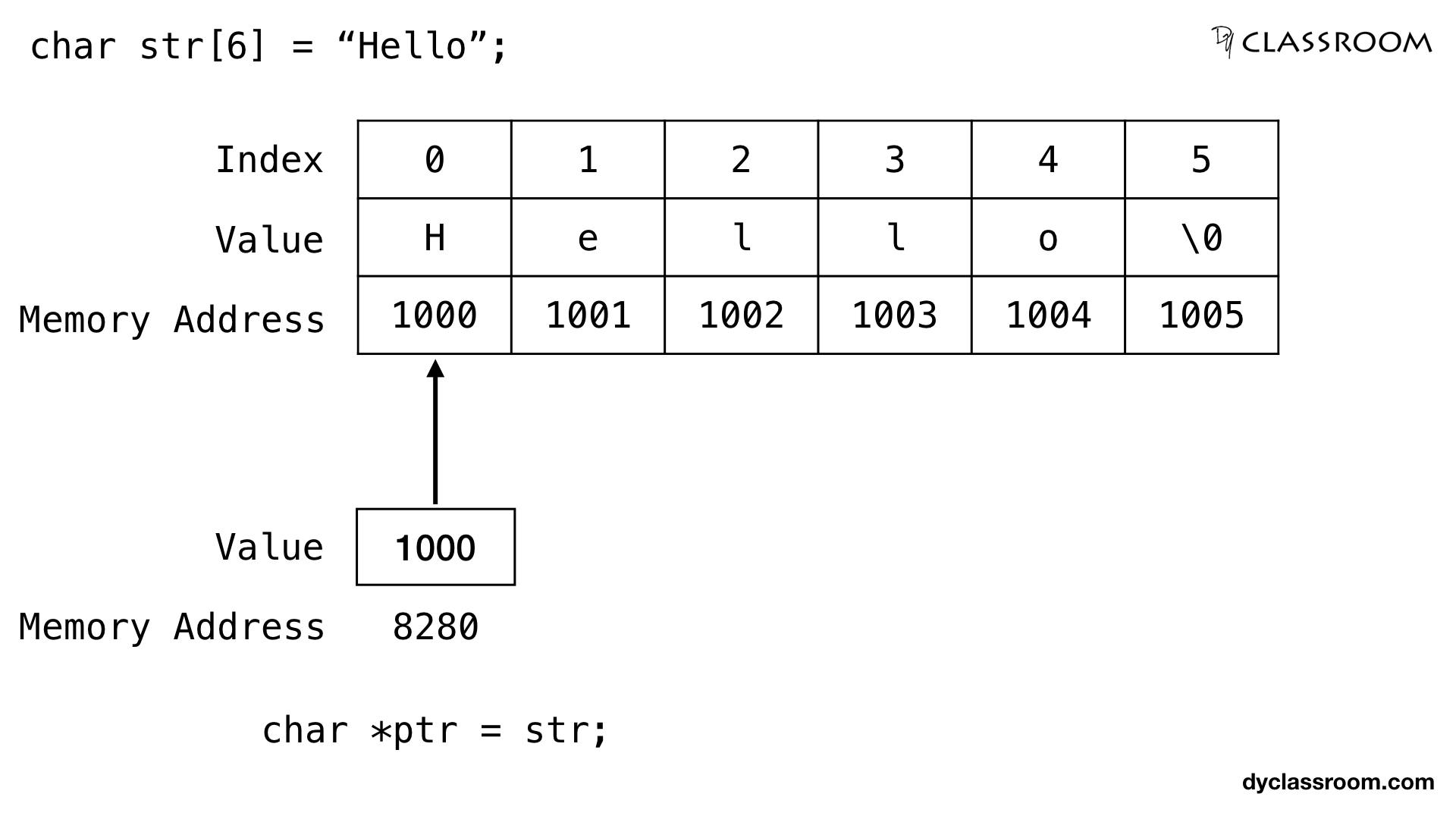# C - Pointers and One Dimensional Array

C ProgrammingIn this tutorial we will learn to work with one dimensional arrays using pointers in C programming language.

We have already learned about how to work with arrays in the Arrays tutorial. So, let us use that knowledge and add pointers to it.

## Create the one dimensional array

To keep things simple we will start by creating an one dimensional character `char` array of size 6.

``````// 1D char array
char str = "Hello";
``````

Three things happens when we create the array.

• 6 blocks of memory locations is allocated for the array.
• The characters of the array are stored in that 6 blocks of memory.
• The variable name `str` points at the first location of the allocated memory locations of the array.In the above image we have the one dimensional character array of size 6 and the memory locations of each element of the array.

## Print the elements of the one dimensional array

First we will using the array index and `for` loop to print the elements of the array.

We will keep printing the characters till we hit the `\0` null character which marks the end of the string.

``````int i;
char str = "Hello";

for (i = 0; str[i] != '\0'; i++) {
printf("%c\n", str[i]);
}
``````

## Creating pointer variable for the one dimensional array

We will create a character pointer `ptr` variable that will hold the address of the character array `str`.

``````// array
char str = "Hello";

// pointer
char *ptr = str;
``````

Note! Array variable points at the beginning address of the allocated memory locations for the array.

In the above code we are storing the beginning address of the array `str` in the pointer variable `ptr`. So, ptr points at the array str.## Incrementing pointer variable

When we increment the pointer variable it points to the next memory location based on the size of the data type.

So, `ptr` character pointer variable is pointing at the first memory location of the one dimensional character array `str`.

And `char` data type is of size 1 byte. So, if the first memory location is 1000 and ptr is initially pointing at that memory location then `ptr++` will make the `ptr` variable point at 1001 memory location.

Similarly, if a pointer variable is of data type `int` and lets say the size of integer is 2 bytes then, if the pointer is initially pointing at the memory location 1000 then after incrementing its value using the `++` operator it will point at the next memory location 1002 holding the next integer data.

## Printing the one dimensional array elements via pointers

We have stored the beginning address of the one dimensional character array `str` in the character pointer variable `ptr`.

Now, using the `++` increment operator we will access the elements of the array.

``````// char array
char str = "Hello";

// pointer
char *ptr = str;

// print the characters
while(*ptr != '\0') {
printf("%c\n", *ptr);
ptr++;
}
``````

### Complete code

``````#include <stdio.h>

int main(void) {

// character array
char str = "Hello";

// pointer ptr
// pointing at the character array str
char *ptr = str;

// print the elements of the array str
while (*ptr != '\0') {
printf("%c\n", *ptr);

// make the pointer ptr point at the
// next memory location
ptr++;
}

printf("End of code\n");

return 0;
}
``````

Output:

``````H
e
l
l
o
End of code
``````

To get the ith element of the one dimensional array we can use the following formula.

``````arr[i] = baseAddress + (i x size_of_data_type)
``````

Where, `baseAddress` is the starting address of the array.

`size_of_data_type` is the size of the data type.

Example, if we are using `char` type then, size is 1 byte. If we are using `int` type then, size is 2 byte.

If we consider the one dimensional character array `str` of size 6.

Then, baseAddress = 1000 and size_of_data_type = 1

``````// address of 0th index element of str
str = baseAddress + (i x size_of_data_type)
= 1000 + (0 x 1)
= 1000

// address of 1st index element of str
str = 1000 + (1 x 1)
= 1001

// address of 5th index element (last element) of str
str = 1000 + (5 x 1)
= 1005
``````